wavethresh
Perform wavelet thresholding (denoising) of the series.
Syntax
Series View: series_name.wavethresh(options)
Options
Basic Options

 threshtype=arg (default = “soft”) Wavelet threshold type: “hard” (hard thresholding), “soft” (soft thresholding). threshlim=arg (default = “universal”) Wavelet threshold limit type: “universal” (universal), “adaptive” (universal adaptive), “minimax” (minimax), “sureshrink” (SureShrink), “fdr” (false discovery rate).If “threshlim=sureshrink”, the grid length may be specified using “ssglen=”.If “threshlim=fdr”, the significance level may be specified using “fdrsig=” wavevar=arg (default = “gauss”) Wavelet coefficient variance method: “mean” (mean absolute deviation), “gauss” (median absolute deviation with Gaussian adjustment), “median” (median absolute deviation), “meanmedian” (mean median absolute deviation). sslen=arg (default = 10) Grid length used in determining the SureShrink limit. fdrsig=arg (default = .05) Significance level as a number between 0 and 1 for false discovery rate limit determination. prompt Force the dialog to appear from within a program. p Print results.
Wavelet Transform Options

 transform=arg (default=“dwt”) Wavelet transform type: “dwt” (discrete wavelet transform – DWT), “modwt” (maximum overlap DWT – MODWT). Note that when performing DWT, if the series length is not dyadic, a dyadic fix may be set with the “fixlen=” option fixlen=arg (default=“mean”) Fix dyadic lengths in DWT: “zeros” (pad remainder with zeros), “mean” (pad remainder with mean of series), “median” (pad remainder with median of series), “shorten” (cut series length to dyadic length preceding series length). maxscale=integer (default = max possible) Maximum scale for wavelet transform. The max possible is obtained as follows. Letdenote the series length and decompose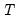into its dyadic component and a remainder: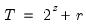,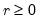. The default maxscale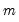is then set with the following rules:DWT: (1) if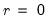then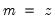, otherwise (2) if expanding the series,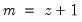and (3) if contracting the series.MODWT:. filter=arg (default=“h”) Wavelet filter class: “h” (Haar), “d” (Daubechies), “la” (least asymmetric).If “filter=h” or “filter=la”, the filter length may be specified using “flen=”.Wavelet filter boundary conditions are specified using the “bound=” option flen=integer Wavelet filter excess length as an even number between 2 and 20.For use when “filter=d” (default= 4) or “filter=la” (default=8). bound=arg (default = “p”) Filter boundary handling: “p” (periodic), “r” (reflective).
Examples
dgp.wavethresh(maxscale=3)
The line above will perform wavelet thresholding on a series called DGP, using a soft threshold and the universal threshold limit. This procedure is also known as VisuShrink. It will do so up to the third wavelet scale and will use the MAD with a Gaussian correction for the measure of loss.
dgp.wavethresh(filter=d, flen=4, maxscale=1, threshtype=hard, threshlim=fdr)
The line above will perform wavelet thresholding using a Daubechies wavelet filter of length 4, up to the first wavelet scale. Furthermore, it will use a hard threshold and the false discovery rate limit with significance level 0.05.
Cross-references
See “Wavelet Analysis” and “Wavelet Threshold (Denoising)” for discussion. See also “Wavelet Objects”.